×
Log in to StudySoup
Get Full Access to Chemistry: A Molecular Approach - 3 Edition - Chapter 1 - Problem 79ae
Join StudySoup for FREE
Get Full Access to Chemistry: A Molecular Approach - 3 Edition - Chapter 1 - Problem 79ae

Already have an account? Login here
×
Reset your password

# AE A 40-lb container of peat moss measures 14 * 20 * 30ISBN: 9780321809247 1

## Solution for problem 79AE Chapter 1

Chemistry: A Molecular Approach | 3rd Edition

• Textbook Solutions
• 2901 Step-by-step solutions solved by professors and subject experts
• Get 24/7 help from StudySoup virtual teaching assistantsChemistry: A Molecular Approach | 3rd Edition

4 5 1 398 Reviews
19
5
Problem 79AE

AE A 40-lb container of peat moss measures 14 * 20 * 30 in. A 40-lb container of topsoil has a volume of 1.9 gal. (a) Calculate the average densities of peat moss and topsoil in units of g/cm3. Would it be correct to say that peat moss is lighter” than topsoil? Explain, (b) How many bags of peat moss are needed to cover an area measuring 15.0 ft * 20.0 ft to a depth of 3.0 in.?

Step-by-Step Solution:

Problem 79AE

AE A 40-lb container of peat moss measures 14 * 20 * 30 in. A 40-lb container of topsoil has a volume of 1.9 gal. (a) Calculate the average densities of peat moss and topsoil in units of g/cm3. Would it be correct to say that peat moss is lighter” than topsoil? Explain, (b) How many bags of peat moss are needed to cover an area measuring 15.0 ft * 20.0 ft to a depth of 3.0 in.?

Step by Step Solution

Step 1 of 2

(a)

From the given,

Peat moss measures =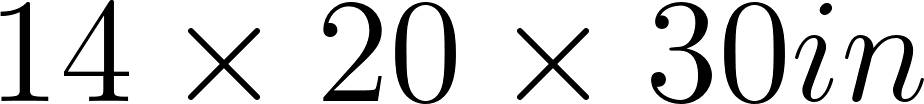Let’s calculate the volume of peat moss;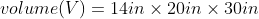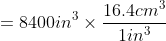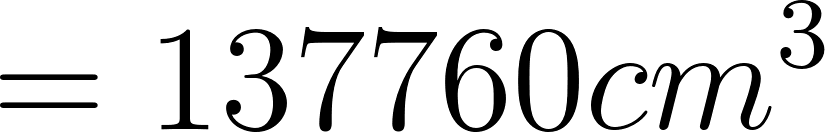Hence, the volume of peat moss is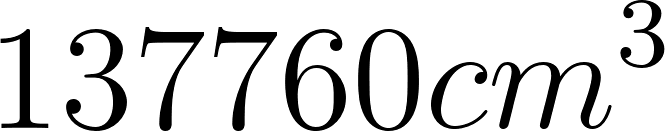.

Let’s calculate the mass of the container of peat moss: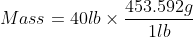Hence, the mass of the container of the peat moss is 18143.68g.

Let’s calculate the density of peat moss;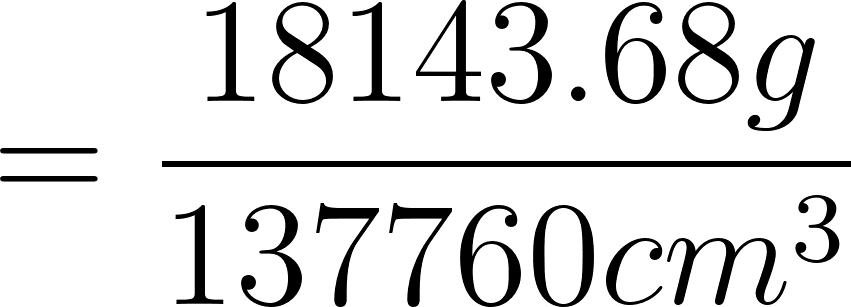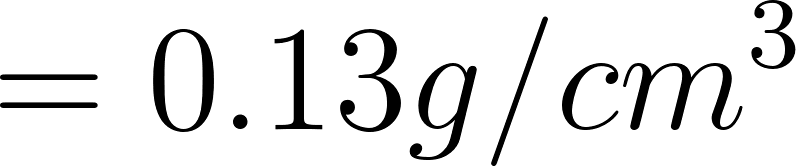Therefore, the density of peat moss is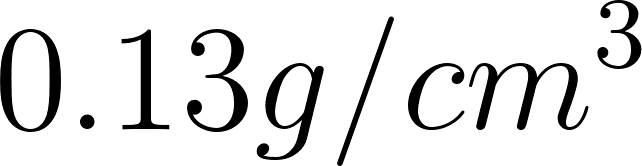.

Let’s calculate the volume of topsoil: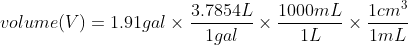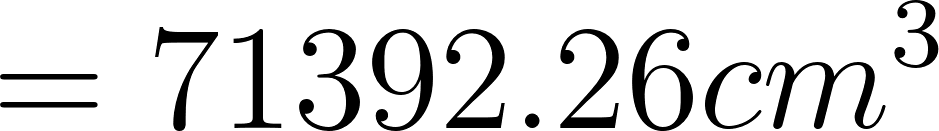Hence, the volume of topsoil is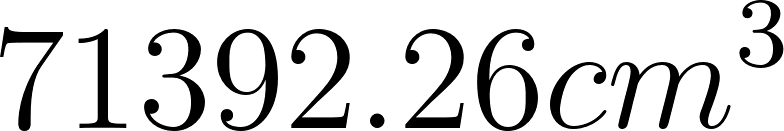.

Let’s calculate the mass of the container of topsoil: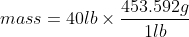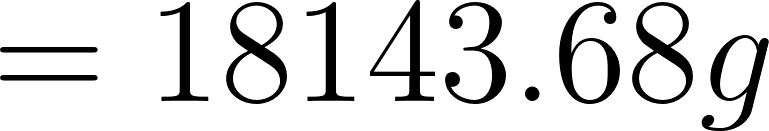Mass of the container of topsoil is 18143.68g.

Let’s calculate the density of topsoil;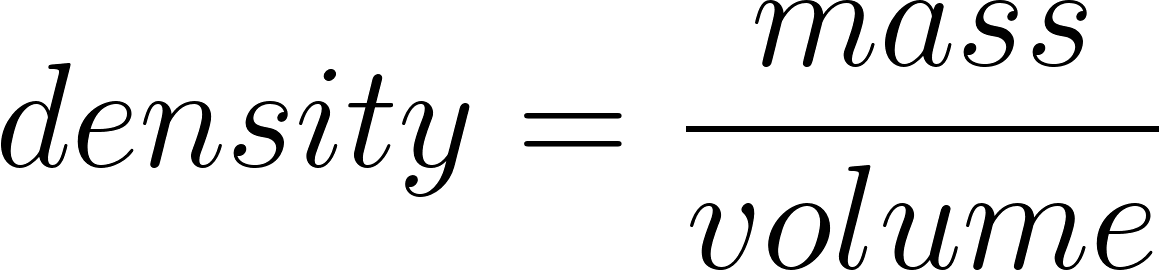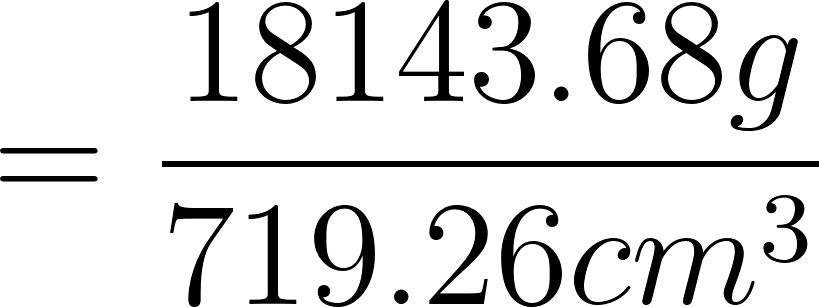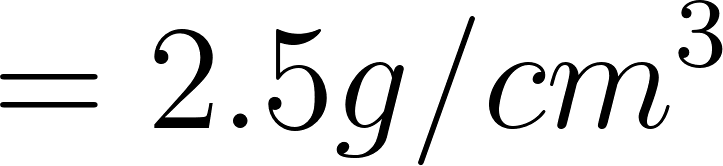Therefore the density of topsoil is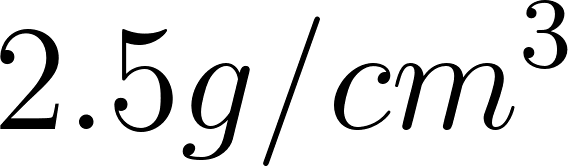.

The density of peat moss is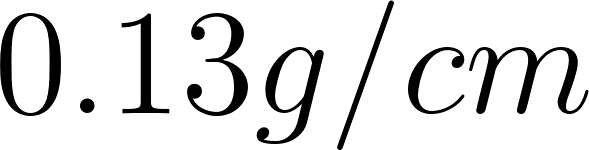and density of topsoil is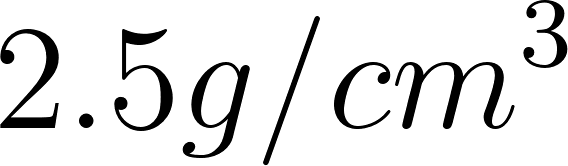. It is not correct to say that the peat moss is lighter than the topsoil. Volume must be specified in order to compare masses.

______________________________________________________________________________

Step 2 of 2

##### ISBN: 9780321809247

This textbook survival guide was created for the textbook: Chemistry: A Molecular Approach, edition: 3. Since the solution to 79AE from 1 chapter was answered, more than 504 students have viewed the full step-by-step answer. The full step-by-step solution to problem: 79AE from chapter: 1 was answered by , our top Chemistry solution expert on 02/22/17, 04:35PM. This full solution covers the following key subjects: moss, peat, topsoil, container, gal. This expansive textbook survival guide covers 82 chapters, and 9454 solutions. The answer to “AE A 40-lb container of peat moss measures 14 * 20 * 30 in. A 40-lb container of topsoil has a volume of 1.9 gal. (a) Calculate the average densities of peat moss and topsoil in units of g/cm3. Would it be correct to say that peat moss is lighter” than topsoil? Explain, (b) How many bags of peat moss are needed to cover an area measuring 15.0 ft * 20.0 ft to a depth of 3.0 in.?” is broken down into a number of easy to follow steps, and 78 words. Chemistry: A Molecular Approach was written by and is associated to the ISBN: 9780321809247.

Unlock Textbook Solution

Enter your email below to unlock your verified solution to:

AE A 40-lb container of peat moss measures 14 * 20 * 30